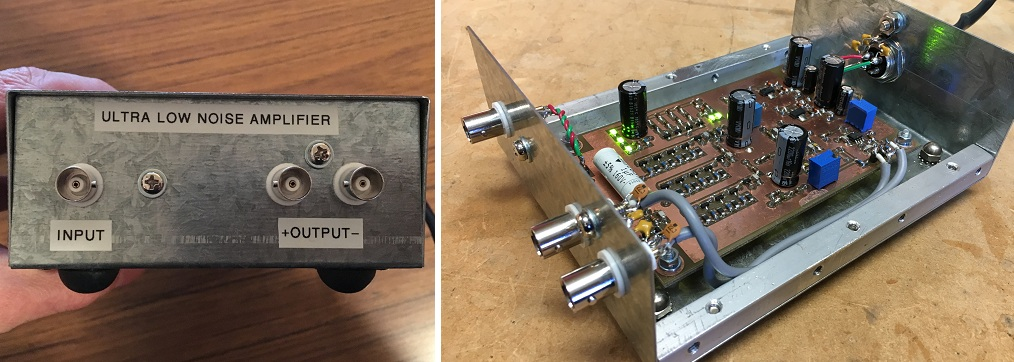# An Ultra Low Noise Measurement Amplifier```In a nutshell:

Voltage noise referred to input (measurement bandwidth = audio A-weighted)	=	< 300 pV SQRT/Hz (JFET = NXP BF862)
Bandwidth 									=	~ 0.1 Hz - ~ 1.5 MHz
Input impedance									=	  1.1 M ohm
Voltage gain (dB fixed)								=	  60 dB (output = single ended)
=	  66 dB (output = balanced)
```